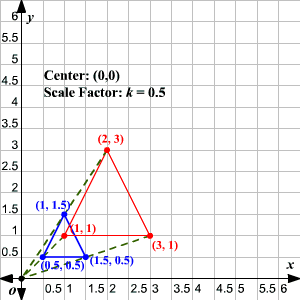# Dilation

A dilation (similarity transformation) is a transformation that changes the size of a figure.  It requires a center point and a scale factor , $k$ .  The value of $k$ determines whether the dilation is an enlargement or a reduction.

If $|k|>1$ , the dilation is an enlargement.

If $|k|<1$ , the dilation is a reduction.

The absolute value of the scale factor determines the size of the new image as compared to the size of the original image.  When $k$ is positive the new image and the original image are on the same side of the center.  When $k$ is negative they are on opposite sides of the center.  The center of a dilation is always its own image.

Dilations preserve angle measure, betweenness of points and collinearity.  It does not preserve distance.  Simply, dilations always produce similar figures .